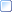# Raw Data

#### EPMA Xtreme Probe

Probe Window Details > Analyze!

### Raw Data

Click this button to see the count rate data for the sample. It will be either beam-corrected data or the raw count data, depending whether the "Use Beam Drift Correction" button in Analysis Options (Analytical menu) is or is not checked (use a Nominal Beam Current of 1 to see counts displayed in counts per second per nA). Note that the Display Count Intensities Unnormalized To Time (in Analyze!) option in Analysis Options will also affect the intensities displayed in the Analyze! window.

The count data will also be printed to the log window. This information includes the average "AVER", standard deviation "SDEV" and the one sigma (ideal) standard deviation, "1SIG", the sigma ratio (standard deviation divided by one sigma), "SIGR" and the standard error "SERR" and percent relative standard deviation "%RSD" which is the standard deviation divided by the average.

The upper data grid will display the count statistics for the sample averaged data including the standard deviation, one sigma, percent relative standard deviation, minimum and maximum values.

The one sigma values represent a predicted standard deviation based on the count rate and the counting time used for the acquisition. It is calculated by dividing the square root of the count rate by the square root of the average counting time. Therefore when counting at one second count times, the one sigma value will be exactly equal to the square root of the count rate. For sample sets using a 10 second counting time, the one sigma value will be smaller than the simple square root by the square root of 10 or approximately 3.162.

Use the one sigma value to evaluate whether a given average count rate represents a homogeneous set of sample data. Standard deviations larger than the one sigma value indicate the possibility of actual compositional variation within the data set. The percent relative standard deviation is simply the standard deviation divided by the average count rate.

Use the Copy buttons to copy this data as tab delimited data to the Windows Clipboard for pasting into another application. The data will automatically formatted as tab delimited text.

The log window will contain additional information including the stage positions for each point in the sample, the on and off-peak positions (absolute and relative offset), the average on-peak and high and low off-peak count times, and for unknown samples only, the Unknown Count Factor multiplier "UNFAC:" and Maximum Counts "MAXCT:", and finally the Time Dependent Intensity (TDI) Calibration Assignment "VOL#:" which is equal to the unknown sample number for the Time Dependent Intensity (TDI) self calibration or equal to another unknown sample number in the case of the assigned Time Dependent Intensity (TDI) calibration.

A new option allows the user to set the Time Dependent Intensity (TDI) element fit type to log-linear (exponential) or to log-quadratic (hyper-exponential). The hyper-exponential fit is useful in cases where the intensity decay is non-exponential. This is usually the case for Na loss in hydrous-alkali glasses where the intensity loss is greater than 50%.

This is followed by the background acquisition type of Mean Atomic Number (MAN), or off-peak (OFF), spectrometer motor number, crystal type, and acquisition order and the MAN or off-peak corrected counts for the sample.

After this, the calculated off-peak counts are also printed out along with the background calculation type (if applicable) of Linear interpolation (LINEAR), high off-peak only (HIGH), low off-peak only (LOW), average of high and low (AVER), slope high (S-HIGH), slope low (S-LOW), exponential interpolation (EXPON) and polynomial (POLY).

More:Summary of Data Printout (standards and unknowns)Example of Data Printout (standards and unknowns)Summary of Data Printout (wavescans)